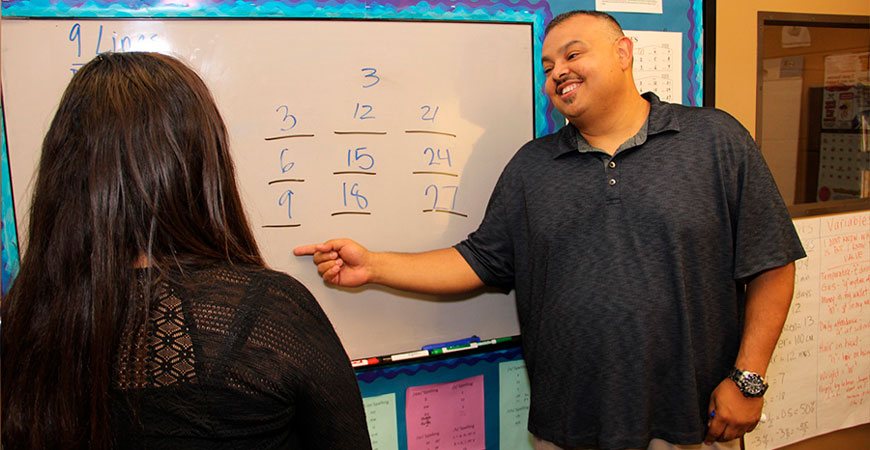## MATHPROGRAM DESCRIPTION

On Cloud Nine® Math: The Lindamood-Bell®, Visualizing and Verbalizing Math Program is a research-based systematic, sequential program centered on stimulation of imagery as fundamental to developing mathematical knowledge from basic number concepts through decimals, fractions, percents and word problems.

On Cloud Nine® Math is designed to assist students who have difficulties learning and mastering basic mathematical computations, concepts and processes. Numeral and concept imagery are developed to allow sensory-cognitive processing to occur, an essential component which enables students to comprehend and use the logic of mathematics in mental and written computations, and to develop successful problem-solving skills. Extensive use of manipulatives and math-related activities allows for development of concretized imagery.

1. Imaging numeral
2. Imaging the number line
4. Subtraction family facts
5. Word problems
6. Place value
7. Jumping
8. Carrying and borrowing
9. Multiplication
10. Division
11. Decimals
12. Fractions
13. Percentages